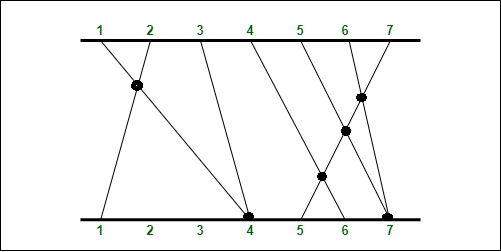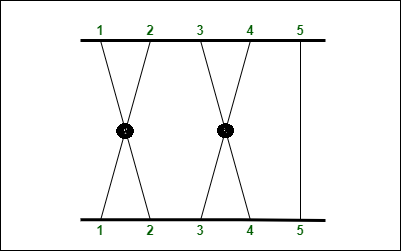GFG App
Open AppBrowser
Continue

# Maximise count of intersections possible from the given endpoints of a line

Given two parallel lines of length N and an array containing total N endpoints in array EndPoints[]. Given that there is a straight line from each i, such that 1 <= i <= N, such that each line should end at any of the EndPoint[j]. The task is to get the count of maximum number of intersections from the lines.

Notice: There should be a total of n lines drawn and every endpoint given in the array should be covered.

Example:

Input: 7
EndPoints[]: {4, 1, 4, 6, 7, 7, 5}
Output: 6input 1

As you can see in the diagram above, a line is drawn from each i (1, 2, 3, 4, 5, 6, 7) and the endpoint is one of the values from EndPoints[] given in the input. It can be proven that there can not be more than 6 intersections in this case.

Input: 5
EndPoints[]: {2, 1, 4, 3, 5}
Output: 2Input 2

### Naive Approach:

Let’s look at two lines from i→EndPoints[i] and j→EndPoints[j].

• If ai<aj, there can never be any intersection.
• If ai>aj, there has to be an intersection.
• If ai=aj, it is possible that there is an intersection or not, depending on how we arrange the lines on the bottom terminal.
• In the last case, if there are multiple lines that go to the same segment ai, we can make all pairs of them cross by arranging the points in which they hit this segment from right to left.

Since we want to maximize the number of intersections, we just need to count the number of pairs (i,j) such that ai≥aj. You can brute force all pairs in O(n2).

Below is the Implementation of the above approach:

## C++

 `#include `   `using` `namespace` `std;`   `void` `maximumIntersection(``int` `EndPoints[], ``int` `n)` `{` `    ``// initializing the total as 0` `    ``int` `total = 0;`   `    ``for` `(``int` `i = 0; i < n; i++) {`   `        ``for` `(``int` `j = i + 1; j < n; j++) {` `            ``// if the previous value is greater than or` `            ``// equal to the current value add it to the` `            ``// answer` `            ``if` `(EndPoints[i] >= EndPoints[j]) {` `                ``total++;` `            ``}` `        ``}` `    ``}` `    ``// Check whether array contains all same element` `    ``int` `first = EndPoints;` `    ``bool` `flag = ``false``;`   `    ``for` `(``int` `i = 1; i < n; i++) {` `        ``// If yes then mark flag as false` `        ``if` `(EndPoints[i] != first) {` `            ``flag = ``false``;` `        ``}` `        ``// Otherwise mark flag as true` `        ``else` `{` `            ``flag = ``true``;` `        ``}` `    ``}`   `    ``if` `(flag == ``true``) {` `        ``cout << ``"Maximum Intersection Possible: "` `             ``<< total / 2 << ``'\n'``;` `    ``}` `    ``else` `{` `        ``cout << ``"Maximum Intersection Possible: "` `<< total` `             ``<< ``'\n'``;` `    ``}` `}`   `// Driver Code` `int` `main()` `{` `    ``int` `X = 7;` `    ``int` `EndPoints[] = { 4, 1, 4, 6, 7, 7, 5 };`   `    ``int` `Y = 5;` `    ``int` `EndPoints2[] = { 2, 1, 4, 3, 5 };`   `    ``int` `Z = 5;` `    ``int` `EndPoints3[] = { 1, 1, 1, 1, 1 };`   `    ``maximumIntersection(EndPoints, X);` `    ``maximumIntersection(EndPoints2, Y);` `    ``maximumIntersection(EndPoints3, Z);` `}`

## Java

 `// Java code to implement the approach`   `import` `java.io.*;` `import` `java.util.*;`   `class` `GFG {`   `static` `void` `maximumIntersection(``int` `EndPoints[], ``int` `n)` `{` `    ``// initializing the total as 0` `    ``int` `total = ``0``;`   `    ``for` `(``int` `i = ``0``; i < n; i++) {`   `        ``for` `(``int` `j = i + ``1``; j < n; j++) {` `            ``// if the previous value is greater than or` `            ``// equal to the current value add it to the` `            ``// answer` `            ``if` `(EndPoints[i] >= EndPoints[j]) {` `                ``total++;` `            ``}` `        ``}` `    ``}` `    ``// Check whether array contains all same element` `    ``int` `first = EndPoints[``0``];` `    ``boolean` `flag = ``false``;`   `    ``for` `(``int` `i = ``1``; i < n; i++) {` `        ``// If yes then mark flag as false` `        ``if` `(EndPoints[i] != first) {` `            ``flag = ``false``;` `        ``}` `        ``// Otherwise mark flag as true` `        ``else` `{` `            ``flag = ``true``;` `        ``}` `    ``}`   `    ``if` `(flag == ``true``) {` `        ``System.out.println( ``"Maximum Intersection Possible: "``+ total / ``2` `);` `    ``}` `    ``else` `{` `        ``System.out.println( ``"Maximum Intersection Possible: "` `+ total);` `    ``}` `}`   `    `  `// Driver code` `public` `static` `void` `main(String[] args)` `{` `    ``int` `X = ``7``;` `    ``int` `EndPoints[] = { ``4``, ``1``, ``4``, ``6``, ``7``, ``7``, ``5` `};`   `    ``int` `Y = ``5``;` `    ``int` `EndPoints2[] = { ``2``, ``1``, ``4``, ``3``, ``5` `};`   `    ``int` `Z = ``5``;` `    ``int` `EndPoints3[] = { ``1``, ``1``, ``1``, ``1``, ``1` `};`   `    ``maximumIntersection(EndPoints, X);` `    ``maximumIntersection(EndPoints2, Y);` `    ``maximumIntersection(EndPoints3, Z);` `}` `}`

## Python3

 `# Python code implementation` `def` `maximum_intersection(endpoints, n):` `    ``# initializing the total as 0` `    ``total ``=` `0` `    ``for` `i ``in` `range``(n):` `        ``for` `j ``in` `range``(i``+``1``, n):` `            ``# if the previous value is greater than or` `            ``# equal to the current value and it to the` `            ``# answer` `            ``if` `endpoints[i] >``=` `endpoints[j]:` `                ``total ``+``=` `1`     `    ``# check whether array contains all same element` `    ``first ``=` `endpoints[``0``]` `    ``flag ``=` `False`   `    ``for` `i ``in` `range``(``1``, n):` `        ``# If yes then mark flag as False` `        ``if` `endpoints[i] !``=` `first:` `            ``flag ``=` `False`   `        ``# Otherwise mark flag as True` `        ``else``:` `            ``flag ``=` `True`   `    ``if` `flag:` `        ``print``(``"Maximum Intersection possible: "``, total``/``/``2``)` `    ``else``:` `        ``print``(``"Maximum Intersection possible: "``, total)`   `# Driver code` `x ``=` `7` `endpoints ``=` `[``4``, ``1``, ``4``, ``6``, ``7``, ``7``, ``5``]`   `y ``=` `5` `endpoints2 ``=` `[``2``, ``1``, ``4``, ``3``, ``5``]`   `z ``=` `5` `endpoints3 ``=` `[``1``, ``1``, ``1``, ``1``, ``1``]`   `maximum_intersection(endpoints, x)` `maximum_intersection(endpoints2, y)` `maximum_intersection(endpoints3, z)`   `# This code is contributed by Prince Kumar`

## C#

 `// C# code to implement the approach` `using` `System;` `public` `class` `GFG {`   `  ``static` `void` `maximumIntersection(``int``[] EndPoints, ``int` `n)` `  ``{`   `    ``// initializing the total as 0` `    ``int` `total = 0;` `    ``for` `(``int` `i = 0; i < n; i++) ` `    ``{`   `      ``for` `(``int` `j = i + 1; j < n; j++)` `      ``{`   `        ``// if the previous value is greater than or` `        ``// equal to the current value add it to the` `        ``// answer` `        ``if` `(EndPoints[i] >= EndPoints[j]) {` `          ``total++;` `        ``}` `      ``}` `    ``}`   `    ``// Check whether array contains all same element` `    ``int` `first = EndPoints;` `    ``bool` `flag = ``false``;`   `    ``for` `(``int` `i = 1; i < n; i++)` `    ``{`   `      ``// If yes then mark flag as false` `      ``if` `(EndPoints[i] != first) {` `        ``flag = ``false``;` `      ``}`   `      ``// Otherwise mark flag as true` `      ``else` `{` `        ``flag = ``true``;` `      ``}` `    ``}`   `    ``if` `(flag == ``true``) {` `      ``Console.WriteLine(` `        ``"Maximum Intersection Possible: "` `        ``+ total / 2);` `    ``}` `    ``else` `{` `      ``Console.WriteLine(` `        ``"Maximum Intersection Possible: "` `+ total);` `    ``}` `  ``}`   `  ``static` `public` `void` `Main()` `  ``{`   `    ``// Code` `    ``int` `X = 7;` `    ``int``[] EndPoints = { 4, 1, 4, 6, 7, 7, 5 };`   `    ``int` `Y = 5;` `    ``int``[] EndPoints2 = { 2, 1, 4, 3, 5 };`   `    ``int` `Z = 5;` `    ``int``[] EndPoints3 = { 1, 1, 1, 1, 1 };`   `    ``maximumIntersection(EndPoints, X);` `    ``maximumIntersection(EndPoints2, Y);` `    ``maximumIntersection(EndPoints3, Z);` `  ``}` `}`   `// This code is contributed by lokesh.`

## Javascript

 `// JavaScript code to implement the approach`   `function` `maximumIntersection( EndPoints, n)` `{` `    ``// initializing the total as 0` `    ``let total = 0;`   `    ``for` `(let i = 0; i < n; i++) {`   `        ``for` `(let j = i + 1; j < n; j++) {` `            ``// if the previous value is greater than or` `            ``// equal to the current value add it to the` `            ``// answer` `            ``if` `(EndPoints[i] >= EndPoints[j]) {` `                ``total++;` `            ``}` `        ``}` `    ``}` `    ``// Check whether array contains all same element` `    ``let first = EndPoints;` `    ``let flag = ``false``;`   `    ``for` `(let i = 1; i < n; i++) {` `        ``// If yes then mark flag as false` `        ``if` `(EndPoints[i] != first) {` `            ``flag = ``false``;` `        ``}` `        ``// Otherwise mark flag as true` `        ``else` `{` `            ``flag = ``true``;` `        ``}` `    ``}`   `    ``if` `(flag == ``true``) {` `       ``console.log( ``"Maximum Intersection Possible: "``+ total / 2 );` `    ``}` `    ``else` `{` `        ``console.log( ``"Maximum Intersection Possible: "` `+ total);` `    ``}` `}`   ` ``// Driver code` `   ``let X = 7;` `   ``let EndPoints = [ 4, 1, 4, 6, 7, 7, 5 ];`   `    ``let Y = 5;` `    ``let EndPoints2 = [ 2, 1, 4, 3, 5 ];`   `    ``let Z = 5;` `    ``let EndPoints3 = [ 1, 1, 1, 1, 1 ];` `    ``// Function call` `    ``maximumIntersection(EndPoints, X);` `    ``maximumIntersection(EndPoints2, Y);` `    ``maximumIntersection(EndPoints3, Z);`

Output

```Maximum Intersection Possible: 6
Maximum Intersection Possible: 2
Maximum Intersection Possible: 5```

Time Complexity: O(N2)
Auxiliary Space: O(1)

### Efficient Approach:

The Basic idea is to use onset template policy based data structure and count frequency of duplicate elements and perform respective operations.

• The above approach can also be optimized if are able to count the number of pairs (i,j) such that ai≥aj in an efficient manner.
•  The ordered set keeps the unique elements in sorted order and provides a number of items strictly smaller than k, which is required in this problem.
`order_of_key(k) : Number of items strictly smaller than k .`
• Thus, we can loop over the input array and add each element one by one and can check order_of_key(element) at each iteration to get the number of elements less than the element and add that to our sum.
• we will have to count the running frequency of each element because we know that equal elements also intersect each other, thus we will be adding the running frequencies to our answer as well.

Below is the Implementation of the above approach:

## C++

 `#include ` `using` `namespace` `std;`   `// Important header files` `#include // Common file` `#include ` `#include // for less` `#include `   `using` `namespace` `std;` `// defining required namespaces for policy based data` `// structure` `using` `namespace` `__gnu_pbds;`   `template` `<``class` `T>` `using` `oset` `    ``= tree, rb_tree_tag,` `           ``tree_order_statistics_node_update>;` `// declaration : oset s;`   `void` `maximumIntersection(``int` `EndPoints[], ``int` `N)` `{` `    ``oset<``int``> st;` `    ``int` `ans = 0;`   `    ``// counting the frequency as there can be duplicate` `    ``// elements as well` `    ``map<``int``, ``int``> mp;`   `    ``for` `(``int` `i = 0; i < N; i++) {` `        ``st.insert(EndPoints[i]);` `        ``// adding mp[EndPoints[i]] because the elements that` `        ``// are equal to EndPoints[i] also intersects each` `        ``// other st.order_of_key(EndPoints[i]) will be` `        ``// returning the number of elements smaller than` `        ``// EndPoints[i]` `        ``ans += st.order_of_key(EndPoints[i])` `               ``+ mp[EndPoints[i]];`   `        ``// adding EndPoints[i] to our map for addressing` `        ``// equal elements` `        ``mp[EndPoints[i]]++;` `    ``}` `    ``// if array contains all similar elements` `    ``if` `(mp.size() == 1) {` `        ``cout << ``"Maximum Intersection Possible: "` `<< ans / 2` `             ``<< ``"\n"``;` `    ``}` `    ``else` `{` `        ``cout << ``"Maximum Intersection Possible: "` `<< ans` `             ``<< ``"\n"``;` `    ``}` `}`   `// Driver Code` `int` `main()` `{` `    ``int` `X = 7;` `    ``int` `EndPoints[] = { 4, 1, 4, 6, 7, 7, 5 };`   `    ``int` `Y = 5;` `    ``int` `EndPoints2[] = { 2, 1, 4, 3, 5 };`   `    ``int` `Z = 5;` `    ``int` `EndPoints3[] = { 1, 1, 1, 1, 1 };`   `    ``maximumIntersection(EndPoints, X);` `    ``maximumIntersection(EndPoints2, Y);` `    ``maximumIntersection(EndPoints3, Z);` `}`

Output

```Maximum Intersection Possible: 6
Maximum Intersection Possible: 2
Maximum Intersection Possible: 5```

Time Complexity: O(N*logN)
Auxiliary Space: O(N)

My Personal Notes arrow_drop_up